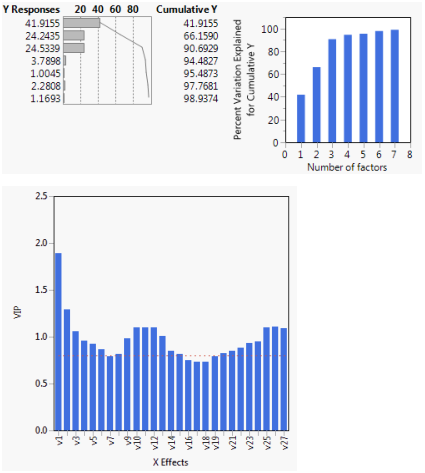Multivariate Methods > Partial Least Squares Models
Publication date: 11/10/2021

# Partial Least Squares Models

##### Develop Models Using Correlations between Ys and Xs

The Partial Least Squares (PLS) platform fits linear models based on factors, namely, linear combinations of the explanatory variables (Xs). These factors are obtained in a way that attempts to maximize the covariance between the Xs and the response or responses (Ys). PLS exploits the correlations between the Xs and the Ys to reveal underlying latent structures.JMP Pro provides additional functionality, enabling you to conduct PLS Discriminant Analysis (PLS-DA), include a variety of model effects, use several validation methods, impute missing data, and obtain bootstrap estimates of the distributions of various statistics.

Partial least squares performs well in situations such as the following, where the use of ordinary least squares does not produce satisfactory results: More X variables than observations; highly correlated X variables; a large number of X variables; several Y variables and many X variables.

Figure 6.1 A Portion of a Partial Least Squares Report